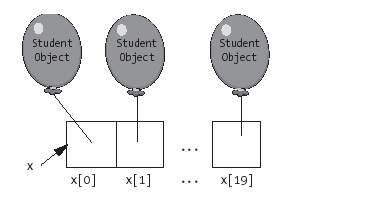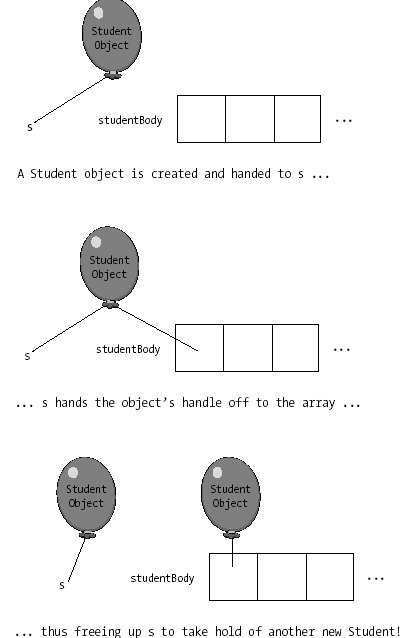FREE BOOK

# Chapter 6: Collections of Objects

Posted by Apress | C# Language December 16, 2008
The properties and behaviors of some common collection types,How collections enable us to model very sophisticated real-world concepts or situations,How we can define our own collection types

Arrays As Simple Collections

One simple type of collection that you may already be familiar with from your work with other programming languages is the array.We can think of an array as a series of compartments, with each compartment sized appropriately for whatever data type the array as a whole is intended to hold. Arrays typically hold items of like type: for example, int(eger)s, or char(acter)s, or, in an OO language, object references: Student objects, or Course objects, or Professor objects, etc.

Declaring and Instantiating Arrays

In C#, arrays are objects (as are all C# collections). The Array class of the System namespace is the basis for all C# arrays. The official C# syntax for declaring that a variable x will serve as a reference to an array containing items of a particular data type is as follows:

datatype[] x;

For example:

int[] x;

which is to be read "int(eger) array x"(or, alternatively, "x is an array of ints").Because C# arrays are objects, they must be instantiated using the new operator; we also specify how many items the array is capable of holding, i.e., its size in terms of its number of compartments, when we first instantiate the array. Here is a code snippet that illustrates the somewhat unusual syntax for constructing an array; in this particular example, we're constructing an array designed to hold Student object references, depicted in Figure 6-3:

// Here, we are instantiating an array object that will be used to store 20
// Student object references, and are maintaining a handle on the array object
// via reference variable x.
Student[] x = new Student;Figure 6-3. Array x is designed to hold up to 20 Student references.

This use of the new operator is unusual, in that we don't see a typical constructor call (with optional arguments being passed in via parentheses) following the new keyword, the way we do when we're constructing other types of objects. Despite its unconventional appearance, however, this line of code is indeed instantiating a new Array object, just the same.

Accessing Individual Array Elements

Individual array elements are accessed by appending square brackets, enclosing the index of the element to be accessed, to the end of the array name. This syntax is known as an element access expression. Note that when we refer to individual items in an array based on their position, or index, relative to the beginning of the array, we start counting at 0. (As it turns out, the vast majority of collection types in C# as well as in other languages are zero-based.) So,the items stored in array Student[] x in our previous example would be individually referenced as x, x, . . . , x.

Consider the following code snippet:

int
[] data = new int;
data = 4;
// setting an element's value
int temp = data; // getting an element's value

In the first line of code, we're declaring and instantiating an int(eger) array of size 3. In the second line of code, we're assigning the int value 4 to the "zeroeth" (first) element of the array. In the last line of code, we're obtaining the value of the second element of the array (element number 1) and assigning it to an int variable named temp.

In the next snippet, we're populating an array named squareRoot of type double to serve as a look-up table of square root values, where the value stored in cell squareRoot[i] represents the square root of i. (We declare the array to be one element larger than we need it to be so that we may skip over the zeroeth cell; for ease of look-up, we want the square root of 1 to be contained in cell 1 of the array, not in cell 0.)

double
[] squareRoot = new double; // we'll effectively ignore cell 0
// Note that we're skipping cell 0.
for
(int i = 1; i <= 10; i++) {
squareRoot[i] = Math.Sqrt(i);
}

Console.WriteLine("The square root of 5 is " + squareRoot);

 The Math.Sqrt() method computes the square root of a double argument passed to the method; we're passing in an int in the preceding example, which automatically gets cast to a double.We'll revisit the Math class again in Chapter 7.

Initializing Array Contents

Values can be assigned to individual elements of an array using indexes as shown earlier, or we can initialize an array with a complete set of values when the array is first instantiated. In the latter case, initial values are provided as a commaseparated list enclosed in braces. This syntax replaces the normal right-hand side of the array instantiation statement. For example, the following code instantiates and initializes a three-element string array:

string
[] names = { "Lisa", "Jackson", "Zachary" };

Note that C# automatically counts the number of initial values that we're providing, and sizes the array appropriately. The preceding approach is much more concise than the equivalent alternative shown here:

string
[] names = new string;
names = "Lisa";
names = "Jackson";
names = "Zachary";

although the result in both cases is the same: the zeroeth (first) element of the array will reference the string "Lisa", the next element will reference "Jackson", and so on.

Note that it isn't possible to "bulk load"an array in this fashion after the array has been instantiated, as a separate line of code; that is, the following
won't work:

string
[] names = new string;
// This next line won't compile.
names = {"Steve", "Jacquie", "Chloe", "Shylow", "Baby Grode" };

If a set of comma-separated initial values aren't provided when an array is first instantiated, the elements of the array are automatically initialized to their zero-equivalent values. For example, int[] data as declared earlier would be initialized to contain 3 integer zeroes (0s), and double[] squareRoot as declared earlier would be initialized to contain 11 floating point zeroes (0.0s). If we declare and instantiate an array intended to hold references to objects, as in

Student[] studentBody = new Student;

then we'd wind up with an Array object containing 100 null values (recall that null, a C# keyword, is the zero equivalent value for an object reference). If we think of an array as a simple type of collection, and we in turn think of a collection as an "egg carton,"then we've just created an empty egg carton with 100 egg compartments, but no "eggs."

Manipulating Arrays of Objects

To fill our Student array with values other than null, we'd have to individually store Student object references in each cell of the array. For example, if we wanted to create brand-new Student objects to store in our array, we may write code as follows:

studentBody = new Student();
studentBody = new Student();
// etc.

or alternatively:

Student s = new Student();
studentBody = s;
// Reuse s!
s = new Student();
studentBody = s;

In the latter example, note that we're "recycling"the same reference variable, s, to create many different Student objects. This works because, after each instantiation, we store a handle on the newly created object in an array compartment, thus allowing s to let go of its handle on that same object, as depicted in Figure 6-4. This technique is used frequently, with all collection types, in virtually all OO programming languages.Figure 6-4. Handing new objects one by one into a collection

When we've created an array to hold objects, as we did for the studentBody array previously, then assuming we've populated the array with object references, an indexed reference to any populated compartment in the array represents an object reference, and can be used accordingly:

studentBody.GetName(); // We're using dot notation to call a method on
// studentBody, the first Student object
// reference in the array.

The syntax of this message may seem a bit peculiar at first, so let's study it a bit more carefully. Since studentBody is declared to be an array capable of holding Student object references, then studentBody[n] represents the contents of the nth compartment of the array-namely, a reference to a Student object! So, the "dot" in the preceding message is separating an expression representing an object reference from the method call being made on that object, and is no different than any of the other dot notation messages that we've seen up until now.

By using a collection such as an array, we don't have to invent a different variable name for each Student object, which means we can step through them all quite easily using a for loop. Here is the syntax for doing so with an array:

// Step through all 100 compartments of the array.

for
(int i = 0; i <= 99; i++) {
// Access the "ith" element of the array -- a Student object (reference) -- so
// that we may print each student's name in turn; in effect, we're printing
// a student roster.
Console.WriteLine(studentBody[i].GetName());
}

 We'll examine a more sophisticated way of stepping through collections in general, using a special type of object called an IEnumerator, in Chapter 13.

Note that we have to take care when stepping through an array to avoid "land mines"due to empty compartments. That is, if we're executing the preceding for loop, but the array isn't completely filled with Student objects, then our invocation of the GetName method will fail as soon as we hit the first empty/null compartment, because in essence we'd be trying to talk to an object that wasn't there! If we modify our code by inserting an if test to guard against null values, however, we'd be OK:

// Step through all 100 compartments of the array.
for
(int i = 0; i <= 99; i++) {
// Avoiding "land mines"!
if
(studentBody[i] != null) {
Console.WriteLine(studentBody[i].GetName());
}
}

 We'll learn in Chapter 13 that this type of failure-namely, attempting to talk to a nonexistent object, or null reference-results in an exception being thrown.

Other Array Considerations

Some other facts concerning C# arrays:

• Once the size of an array has been declared, its size can't be changed; This can become an issue in situations where we don't know what the size of an array should be at the time that we're declaring it. For this reason, arrays aren't always the best choice of collection type for a given application, as we'll discuss later in this chapter.

• We can't mix and match data types in an array; we're constrained to inserting values whose data type matches the type with which the array was first declared. For instance, we can't assign a string as an element of an integer array. The only exception is that if we can cast some value of type A into type B, then we can of course make such an assignment. For example, if we wish to assign the value of a double variable to an element of a float array, we would cast the variable into a float and then assign it to the array element as shown here:

float[] a = new float;
double d = 10.37;
a = (float) d; // note cast